# Touch-Tone Telephone Dialing8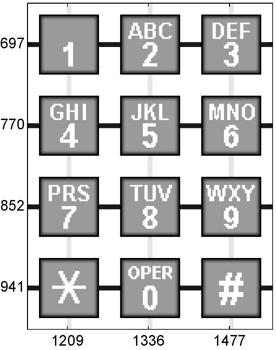We all use Fourier analysis every day without even knowing it. Cell phones, disc drives, DVDs, and JPEGs all involve fast finite Fourier transforms. This post, which describes touch-tone telephone dialing, is the first of three posts about the computation and interpretation of FFTs. The posts are adapted from chapter 8 of my book, Numerical Computing with MATLAB . ... read more >>

# Complete Pivoting and Hadamard Matrices1

For several years we thought Hadamard matrices showed maximum element growth for Gaussian elimination with complete pivoting. We were wrong. ... read more >>

# Gaussian Elimination with Partial Pivoting1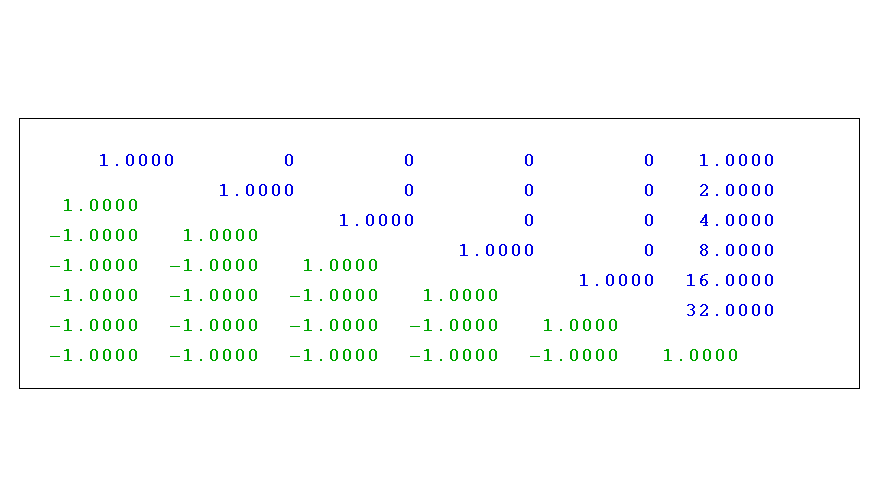In rare cases, Gaussian elimination with partial pivoting is unstable. But the situations are so unlikely that we continue to use the algorithm as the foundation for our matrix computations.... read more >>

# Floating Point Denormals, Insignificant But Controversial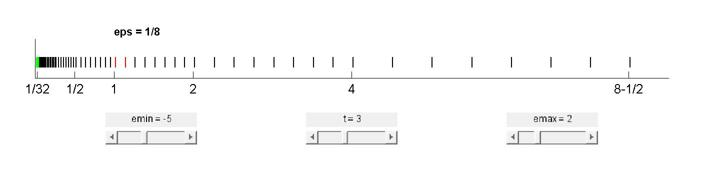Denormal floating point numbers and gradual underflow are an underappreciated feature of the IEEE floating point standard. Double precision denormals are so tiny that they are rarely numerically significant, but single precision denormals can be in the range where they affect some otherwise unremarkable computations. Historically, gradual underflow proved to be very controversial during the committee deliberations that developed the standard. ... read more >>

# Floating Point Numbers5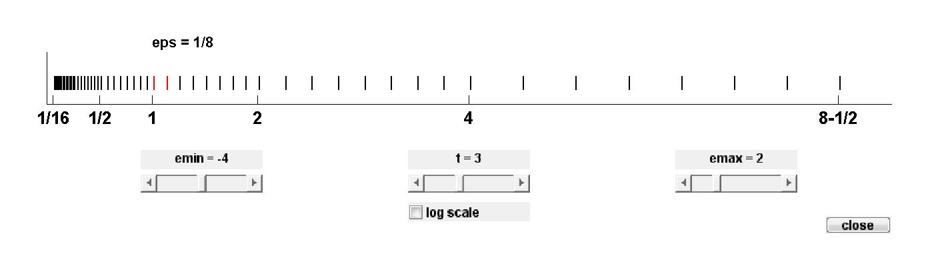This is the first part of a two-part series about the single- and double precision floating point numbers that MATLAB uses for almost all of its arithmetic operations. (This post is adapted from section 1.7 of my book Numerical Computing with MATLAB, published by MathWorks and SIAM.) ... read more >>

# Ordinary Differential Equations, Stiffness3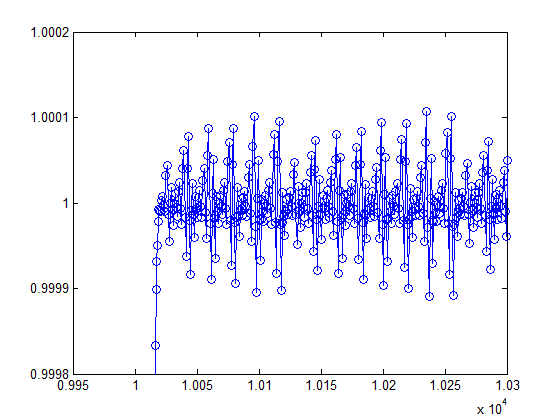# Ordinary Differential Equation Solvers ODE23 and ODE454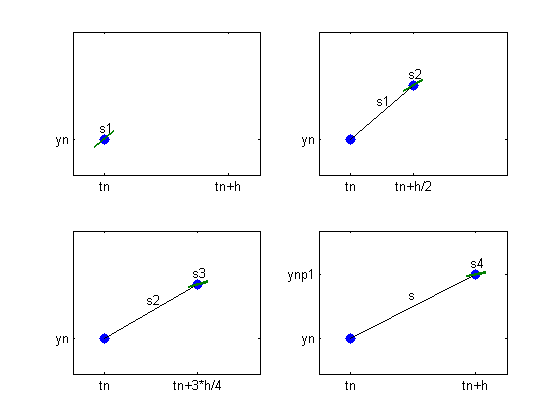The functions ode23 and ode45 are the principal MATLAB and Simulink tools for solving nonstiff ordinary differential equations.... read more >>

# Ordinary Differential Equation Suite4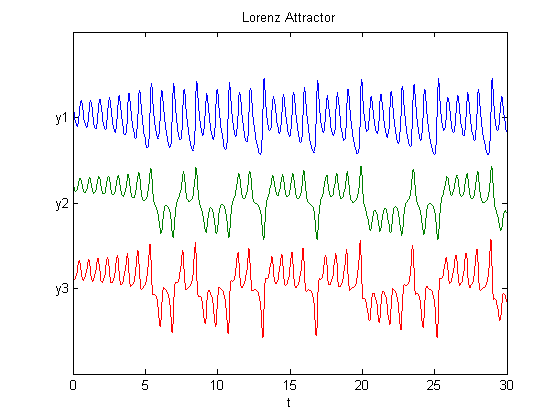MATLAB and Simulink have a powerful suite of routines for the numerical solution of ordinary differential equations. Today's post offers an introduction. Subsequent posts will examine several of the routines in more detail.... read more >>

# Fiedler Companion Matrix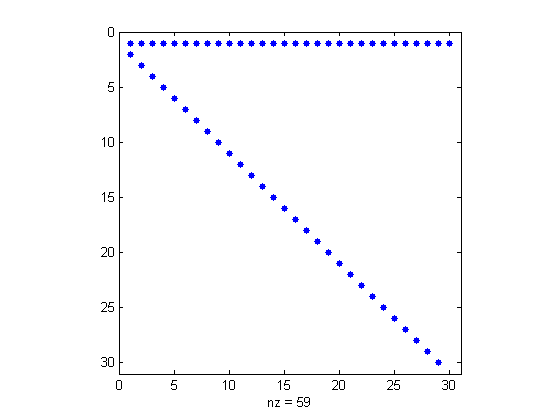The Fiedler companion matrix distributes the coefficients of a polynomial along the diagonals of an elegant pentadiagonal matrix whose eigenvalues are equal to the zeros of the polynomial.... read more >>

# Complex Step Differentiation3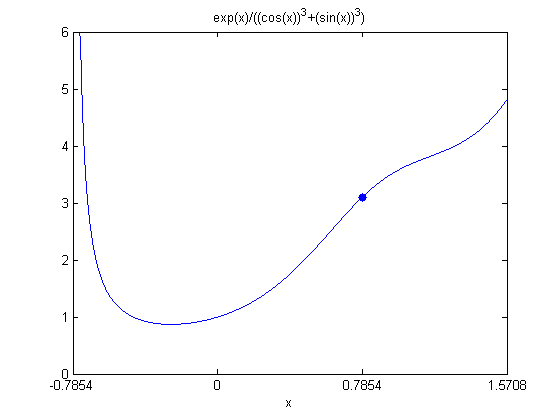Complex step differentiation is a technique that employs complex arithmetic to obtain the numerical value of the first derivative of a real valued analytic function of a real variable, avoiding the loss of precision inherent in traditional finite differences.... read more >>